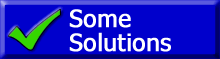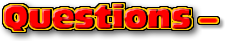ChaptersThis text is meant to accompany class discussions. It is not everything there is to know about the basics of electricity. It is meant as a  prep for class. More detailed notes and examples are given in the class notes, presentations, and demonstrations (click here.)
 Table of Contents Page 0 Objectives 0 Introduction 1 Current 2 Resistance 2 Resistance and Resistivity 2 Resistance and Temperature 3 Ohm's Law 3 Voltage and Potential Difference 3 Current Types (a.c. - d.c.) 4 Power 4 Thermal Power Loss 5 Watts versus Volt•Amps in Appliances 5 Battery Life 5 How Appliances Get Their Energy 5 Calculating the Cost of Electricity

 ResourcesObjectives

Students should be able to:

1. Write all the chapter’s formulae from memory
2. Define current
3. Relate current charge and time
4. Define "resistivity" in a sentence.
5. Define resistance.
6. Describe the factors that affect resistance.
7. Calculate how temperature can effect resistance.
8. Define all the S.I. units of each variable.
9. Define Ohm’s Law
10. Calculate the power used by an appliance.
11. Use the power relationships to solve problems.
12. Define a.c. and d.c. and give examples of each.
13. Identify the current graph associated with an a.c. or d.c. current.
14. Describe, simplistically, what a battery is.
15. Calculate the charge value of a battery in the consumer units of Ah or mAh.
16. Calculate the life of a battery when it is connected to an electrical device.
17. Calculate the cost of running a certain appliance that is plugged into the standard a.c. outlet.
18. Calculate how long an appliance needs to run to use up a specified amount of money.
19. Describe how a household circuit works in relation to a circuit breaker or fuse.
20. Describe how a replaceable fuse works.
21. Describe what each “hole” in a household outlet “does” or means.Click for the questions that go with this reading Introduction

"Electricity" describes the flow of moving charges. In the previous chapter on electrostatics, collecting and placing charges was studied. The role of the electric field is defined in a circuit at providing the force needed to push charged energy carriers along wires. These charged carriers deliver a set amount of energy to a device such as a flashlight or MP3 player.

It is important to note that charges, such as electrons or positive ions, are not consumed by an electronic device. These charges deliver some of their energy to an electronic device. If these charges come from a 6 Volt battery, then each charge will lose 6 electronVolts of energy. But the energy of each charge as it enters the device is not 6 eV's. It may be 10, 20, or 3000 eV's. What each charge does the same is to lose the number of eV's equal to a the battery's voltage.

The work of the electric field is described in various ways. This unit will define those ways and describe some applications of these descriptions.

by Tony Wayne ...(If you are a teacher, please feel free to use these resources in your teaching.)